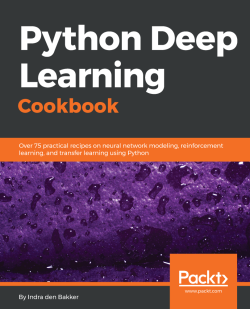Python Deep Learning Cookbook4.5 (8 reviews total)
By Indra den Bakker
• \$5/mo for 5 months Subscribe Access now
• \$35.99 eBook

• Constantly updated with 100+ new titles each month
• Breadth and depth in over 1,000+ technologies

Deep Learning is revolutionizing a wide range of industries. For many applications, deep learning has proven to outperform humans by making faster and more accurate predictions. This book provides a top-down and bottom-up approach to demonstrate deep learning solutions to real-world problems in different areas. These applications include Computer Vision, Natural Language Processing, Time Series, and Robotics.

The Python Deep Learning Cookbook presents technical solutions to the issues presented, along with a detailed explanation of the solutions. Furthermore, a discussion on corresponding pros and cons of implementing the proposed solution using one of the popular frameworks like TensorFlow, PyTorch, Keras and CNTK is provided. The book includes recipes that are related to the basic concepts of neural networks. All techniques s, as well as classical networks topologies. The main purpose of this book is to provide Python programmers a detailed list of recipes to apply deep learning to common and not-so-common scenarios.

Publication date:
October 2017
Publisher
Packt
Pages
330
ISBN
9781787125193

Chapter 1. Programming Environments, GPU Computing, Cloud Solutions, and Deep Learning Frameworks

This chapter focuses on technical solutions to set up popular deep learning frameworks. First, we provide solutions to set up a stable and flexible environment on local machines and with cloud solutions. Next, all popular Python deep learning frameworks are discussed in detail:

• Setting up a deep learning environment
• Launching an on Amazon Web Services (AWS)
• Launching an on Google Cloud Platform (GCP)
• Installing CUDA and cuDNN
• Installing Anaconda and libraries
• Connecting with Jupyter Notebook on a server
• Building state-of-the-art, production-ready models with TensorFlow
• Intuitively building networks with Keras
• Using PyTorch's dynamic computation graphs for RNNs
• Implementing high-performance models with CNTK
• Building efficient models with MXNet
• Defining networks using simple and efficient code with Gluon

Introduction

The recent advancements in deep learning can be, to some extent, attributed to the advancements in computing power. The increase in computing power, more specifically the use of GPUs for processing data, has contributed to the leap from shallow neural networks to deeper neural networks. In this chapter, we lay the groundwork for all following chapters by showing you how to set up stable environments for different deep learning frameworks used in this cookbook. There are many open source deep learning frameworks that are used by researchers and in the industry. Each framework has its own benefits and most of them are supported by some big tech company.

By following the steps in this first chapter carefully, you should be able to use local or cloud-based CPUs and GPUs to leverage the recipes in this book. For this book, we've used Jupyter Notebooks to execute all code blocks. These notebooks provide interactive feedback per code block in such a way that it's perfectly suited for storytelling.

The download links in this recipe are intended for an Ubuntu machine or server with a supported NVIDIA GPU. Please change the links and filenames accordingly if needed. You are free to use any other environment, package managers (for example, Docker containers), or versions if needed. However, additional steps may be required.

Setting up a deep learning environment

Before we get with training deep learning models, we need to set up our deep learning environment. While it is possible to run deep learning models on CPUs, the speed achieved with GPUs is significantly higher and necessary when running deeper and more complex models.

How to do it...

1. First, you need to check whether you have to a CUDA-enabled NVIDIA GPU on your local machine. You can check the overview at https://developer.nvidia.com/cuda-gpus.
2. If your GPU is listed on that page, you can continue installing CUDA and cuDNN if you haven't done that already. Follow the steps in the Installing CUDA and cuDNN section.
1. If you don't have to an NVIDIA GPU on your local machine, you can decide to use a cloud solution. Follow the steps in the Launching a cloud solution section.

Launching an instance on Amazon Web Services (AWS)

Amazon Web Services (AWS) is the most cloud solution. If you don't have access to a local GPU or if you prefer to use a server, you can set up an EC2 on AWS. In this recipe, we provide steps to launch a GPU-enabled server.

Before we move on with this recipe, we assume that you already have an account on Amazon AWS and that you are familiar with its platform and the accompanying costs.

How to do it...

1. Make sure the region you want to work in gives access to P2 or G3 instances. These instances include NVIDIA K80 GPUs and NVIDIA Tesla M60 GPUs, respectively. The K80 GPU is faster and has more GPU memory than the M60 GPU: 12 GB versus 8 GB.

Note

While the NVIDIA K80 and M60 GPUs are powerful GPUs for running deep learning models, these should not be considered state-of-the-art. Other faster GPUs have already been launched by NVIDIA and it takes some time before these are added to cloud solutions. However, a big advantage of these cloud machines is that it is straightforward to scale the number of GPUs attached to a machine; for example, Amazon's p2.16xlarge instance has 16 GPUs.

1. There are two when launching an AWS instance. Option 1: You build everything from scratch. Option 2: You use a preconfigured Amazon Machine Image (AMI) from the  marketplace. If you choose option 2, you will have to pay costs. For an example, see this AMI at https://aws.amazon.com/marketplace/pp/B06VSPXKDX.
2. Amazon provides a and up-to-date overview of steps to launch the deep learning AMI at https://aws.amazon.com/blogs/ai/get-started-with-deep-learning-using-the-aws-deep-learning-ami/.
3. If you want to build the server from scratch, launch a P2 or G3 instance and follow the steps under the Installing CUDA and cuDNN and Installing Anaconda and Libraries recipes.
4. Always make sure you stop the running instances when you're done to prevent unnecessary costs.

Note

A good option to save costs is to use AWS Spot instances. This allows you to bid on spare Amazon EC2 computing capacity.

Launching an instance on Google Cloud Platform (GCP)

Another popular cloud provider is Google. Its Google Cloud Platform (GCP) is getting more and has as a major benefit—it includes a newer GPU type, NVIDIA P100, with 16 GB of GPU memory. In this recipe, we provide the steps to launch a GPU-enabled compute machine.

Before with this recipe, you should be with GCP and its cost structure.

How to do it...

1. You need to request an increase in the GPU quota before you launch a compute instance with a GPU for the first time. Go to https://console.cloud.google.com/projectselector/iam-admin/quotas.
2. First, select the project you want to use and apply the Metric and Region filters accordingly. The GPU instances should show up as follows: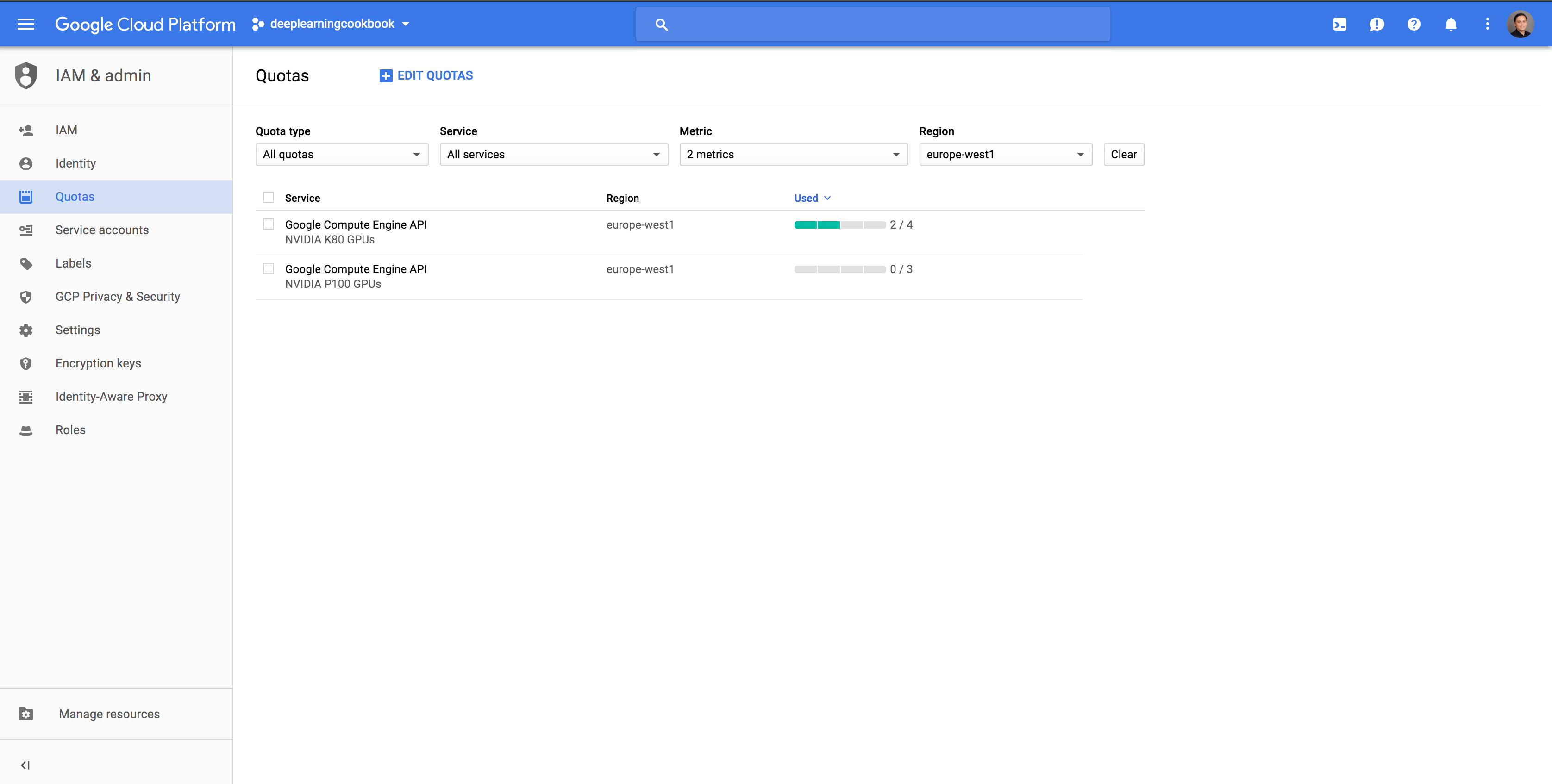Figure 1.1: Google Cloud Platform dashboard for increasing the GPU quotas

1. Select the quota you want to change, click on EDIT QUOTAS, and follow the steps.
2. You will get an e-mail confirmation when your quota has been increased.
3. Afterwards, you can create a GPU-enabled machine.
4. When launching a machine, make sure you tick the Allow HTTP traffic and Allow HTTPs traffic boxes if you want to use a Jupyter notebook.

Installing CUDA and cuDNN

This part is if you want to leverage GPUs for deep learning. The CUDA toolkit is specially designed for GPU-accelerated applications, where the compiler is optimized for using math operations. In addition, the cuDNN library—short for CUDA Deep Neural Network library—is a library that accelerates deep learning routines such as convolutions, pooling, and activation on GPUs.

Make sure you've registered for Nvidia's Accelerated Computing Developer Program at https://developer.nvidia.com/cudnn before starting with this recipe. Only after will you have access to the files needed to install the cuDNN library.

How to do it...

1. Next, we unpack the file and update all all packages in the package lists. Afterwards, we remove the downloaded file:
sudo dpkg -i cuda-repo-ubuntu1604_8.0.61-1_amd64.deb
sudo apt-get update
rm cuda-repo-ubuntu1604_8.0.61-1_amd64.deb
1. Now, we're ready to install CUDA with the following command:
sudo apt-get install cuda-8-0
1. Next, we need to set the environment variables and add them to the shell script .bashrc:
echo 'export CUDA_HOME=/usr/local/cuda' >> ~/.bashrc
echo 'export PATH=\$PATH:\$CUDA_HOME/bin' >> ~/.bashrc
echo 'export LD_LIBRARY_PATH=\$LD_LIBRARY_PATH:\$CUDA_HOME/lib64' >> ~/.bashrc
1. Make sure to reload the shell script afterwards with the following command:
source ~/.bashrc
1. You can check whether the 8.0 driver and toolkit are correctly using the following commands in your terminal:
nvcc --version
nvidia-smi

The output of the last command should look something like this: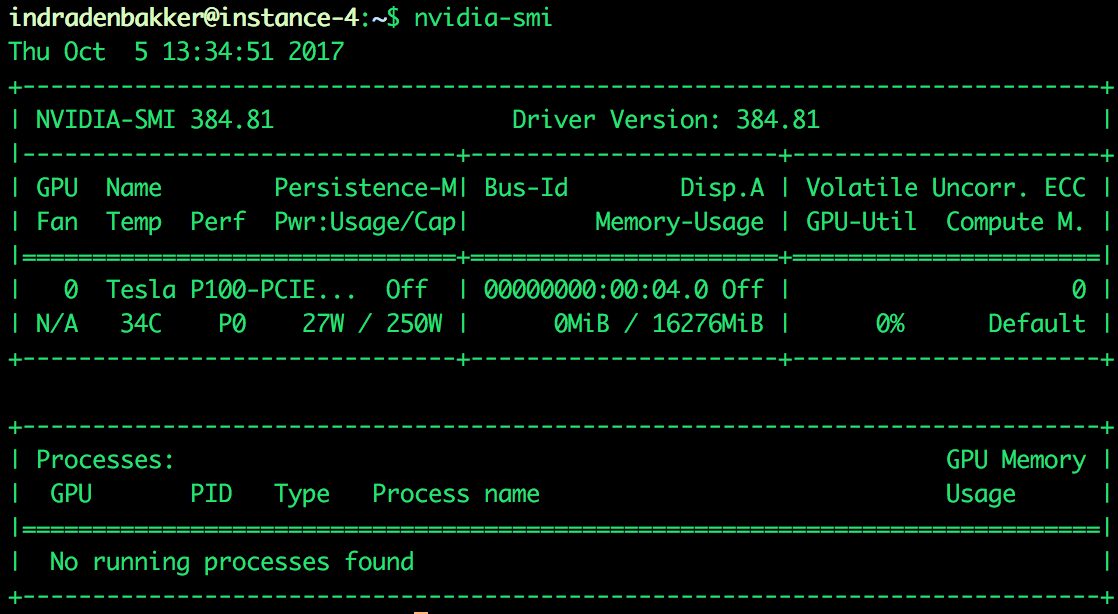Figure 1.2: Example output of nvidia-smi showing the connected GPU

1. Here, we can see that an NVIDIA P100 GPU with 16 GB of memory is correctly connected and ready to use.
1. We are now ready to install cuDNN. Make sure the NVIDIA cuDNN file is available on the machine, for example, by copying from your local machine to the server if needed. For Google cloud compute engine (make sure you've set up gcloud and the project and zone are set up correctly), you can use the following command (replace local-directory and instance-name with your own settings):
gcloud compute scp local-directory/cudnn-8.0-linux-x64-v6.0.tgz instance-name
1. First we the file before copying to the right as root:
cd
tar xzvf cudnn-8.0-linux-x64-v6.0.tgz
sudo cp cuda/lib64/* /usr/local/cuda/lib64/
sudo cp cuda/include/cudnn.h /usr/local/cuda/include/
1. To clean up our space, we can remove the files we've used for installation, as follows:
rm -rf ~/cuda
rm cudnn-8.0-linux-x64-v5.1.tgz

Installing Anaconda and libraries

One of the most popular environment managers for users is Anaconda. With Anaconda, it's straightforward to set up, switch, and delete environments. Therefore, one can run Python 2 and Python 3 on the same machine and switch between different installed versions of installed libraries if needed. In this book, we purely focus on Python 3 and every recipe can be run within one environment: environment-python-deep-learning-cookbook.

How to do it...

curl -O https://repo.continuum.io/archive/Anaconda3-4.3.1-Linux-x86_64.sh
1. Next, run the bash script (if necessary, adjust the filename accordingly):
bash Anaconda3-4.3.1-Linux-x86_64.sh

Follow all prompts and choose 'yes' when you're asked to to add the PATH to the .bashrc file (the default is 'no').

source ~/.bashrc
1. Now, let's set up an Anaconda environment. Let's start with copying the files from the GitHub repository and opening the directory:
cd Python-Deep-Learning-Cookbook-Kit
1. Create the environment with the following command:
conda env create -f environment-deep-learning-cookbook.yml
1. This creates an named environment-deep-learning-cookbook and installs all libraries and dependencies included in the .yml file. All used in this book are included, for example, NumPy, OpenCV, Jupyter, and scikit-learn.
2. Activate the environment:
source activate environment-deep-learning-cookbook
1. You're now ready to run Python. Follow the next recipe to install Jupyter and the deep learning frameworks used in this book.

Connecting with Jupyter Notebooks on a server

As mentioned in the introduction, Notebooks have gained a lot of traction in the last couple of years. Notebooks are an intuitive tool for running blocks of code. When creating the Anaconda environment in the Installing Anaconda and Libraries recipe, we included Jupyter in our list of libraries to install.

How to do it...

1. If you haven't installed Jupyter yet, you can use the following command in your activated Anaconda environment on the server:
conda install jupyter
1. Next, we move back to the terminal on our local machine.
1. One option is to access the Notebook running on a server using SSH-tunnelling. For example, when using Google Cloud Platform:
gcloud compute ssh --ssh-flag="-L 8888:localhost:8888"  --zone "europe-west1-b" "instance-name"

You're now logged in to the server and port 8888 on your local machine will forward to the server with port 8888.

1. Make sure to activate the correct Anaconda environment before proceeding (adjust the name of your environment accordingly):
source activate environment-deep-learning-cookbook
1. You can create a dedicated directory for your Jupyter notebooks:
mkdir notebooks
cd notebooks
1. You can now start the Jupyter environment as follows:
jupyter notebook

This will start Jupyter Notebook on your server. Next, you can go to your local browser and access the notebook with the link provided after starting the notebook, for example, http://localhost:8888/?token=1fa4e9aea99cd7be2b974557eee3d344ca3c992f5861834f.

Building state-of-the-art, production-ready models with TensorFlow

One of the most—if not the most—popular frameworks at the moment is TensorFlow. The framework is created, maintained, and used internally by Google. This general open source framework can be used for any numerical computation by using data flow graphs. One of the biggest advantages of using is that you can use the same code and deploy it on your local CPU, GPU, or device. TensorFlow can also be used to run your deep learning model across multiple GPUs and CPUs.

How to do it...

1. First, we will show how to install from your terminal (make sure that you adjust the link to the wheel for your and Python accordingly):

This will install the GPU-enabled version of TensorFlow and the correct dependencies.

1. You can now import the TensorFlow library into your Python environment:
import tensorflow as tf
1. To provide a dummy dataset, we will use numpy and the following code:
import numpy as np
x_input = np.array([[1,2,3,4,5]])
y_input = np.array([])
1. When defining a TensorFlow model, you cannot feed the data directly to your model. You should create a placeholder that acts like an entry point for your data feed:
x = tf.placeholder(tf.float32, [None, 5])
y = tf.placeholder(tf.float32, [None, 1])
1. Afterwards, you apply some operations to the placeholder with some variables. For example:
W = tf.Variable(tf.zeros([5, 1]))
b = tf.Variable(tf.zeros())
y_pred = tf.matmul(x, W)+b
1. Next, define a loss function as follows:
loss = tf.reduce_sum(tf.pow((y-y_pred), 2))
1. We need to specify the optimizer and the variable that we want to minimize:
1. In TensorFlow, it's important that you initialize all variables. Therefore, we create a variable called init:
init = tf.global_variables_initializer()

We should note that this command doesn't initialize the variables yet; this is done when we run a session.

1. Next, we create a session and run the training for 10 epochs:
sess = tf.Session()
sess.run(init)

for i in range(10):
feed_dict = {x: x_input, y: y_input}
sess.run(train, feed_dict=feed_dict)
1. If we also want to extract the costs, we can do so by adding it as follows:
sess = tf.Session()
sess.run(init)

for i in range(10):
feed_dict = {x: x_input, y: y_input}
_, loss_value = sess.run([train, loss], feed_dict=feed_dict)
print(loss_value)
1. If we want to use multiple GPUs, we should specify this explicitly. For example, take this part of code from the TensorFlow documentation:
c = []
for d in ['/gpu:0', '/gpu:1']:
with tf.device(d):
a = tf.constant([1.0, 2.0, 3.0, 4.0, 5.0, 6.0], shape=[2, 3])
b = tf.constant([1.0, 2.0, 3.0, 4.0, 5.0, 6.0], shape=[3, 2])
c.append(tf.matmul(a, b))
with tf.device('/cpu:0'):
# Creates a session with log_device_placement set to True.
sess = tf.Session(config=tf.ConfigProto(log_device_placement=True))
# Runs the op.
print(sess.run(sum))

As you can see, this gives a lot of flexibility in how the computations are handled and by which device.

Note

This is just a brief introduction to how TensorFlow works. The granular level of model implementation gives the user a lot of flexibility when implementing networks. However, if you're new to neural networks, it might be overwhelming. That is why the Keras framework--a wrapper on top of TensorFlow—can be a good alternative for those who want to start building neural networks without getting too much into the details. Therefore, in this book, the first few chapters will mainly focus on Keras, while the more advanced chapters will include more recipes that use other frameworks such as TensorFlow.

Intuitively building networks with Keras

Keras is a deep learning framework that is known and adopted by deep learning engineers. It provides a wrapper around the TensorFlow, CNTK, and the Theano frameworks. This wrapper you gives the ability to easily create deep learning models by stacking different types of layers. The power of Keras lies in its simplicity and readability of the code. If you want to use multiple GPUs during training, you need to set the devices in the same way as with TensorFlow.

How to do it...

1. We start by installing Keras on our local Anaconda environment as follows:
conda install -c conda-forge keras

Make sure your deep learning environment is activated before executing this command.

1. Next, we import keras library into our Python environment:
from keras.models import Sequential
from keras.layers import Dense

This command outputs the used by Keras. By default, the TensorFlow framework is used: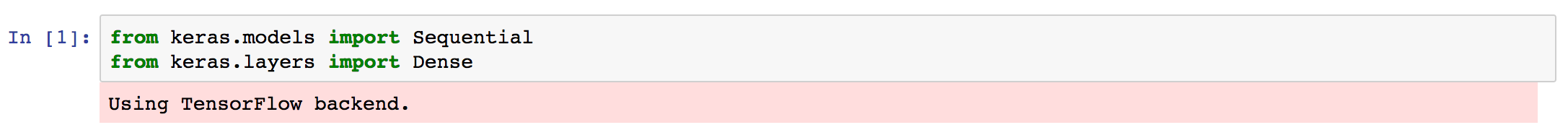Figure 1.3: Keras prints the backend used

1. To provide a dummy dataset, we will use numpy and the following code:
import numpy as np
x_input = np.array([[1,2,3,4,5]])
y_input = np.array([])
1. When using sequential mode, it's straightforward to stack multiple layers in Keras. In this example, we use one hidden layer with 32 units and an output layer with one unit:
model = Sequential()
1. Next, we need to compile our model. While compiling, we can set different settings such as loss function, optimizer, and metrics:
model.compile(loss='mse',
optimizer='sgd',
metrics=['accuracy'])
1. In Keras, you can easily print a summary of your model. It will also show the number of parameters within the defined model:
model.summary()

In the following figure, you can see the summary of our build model: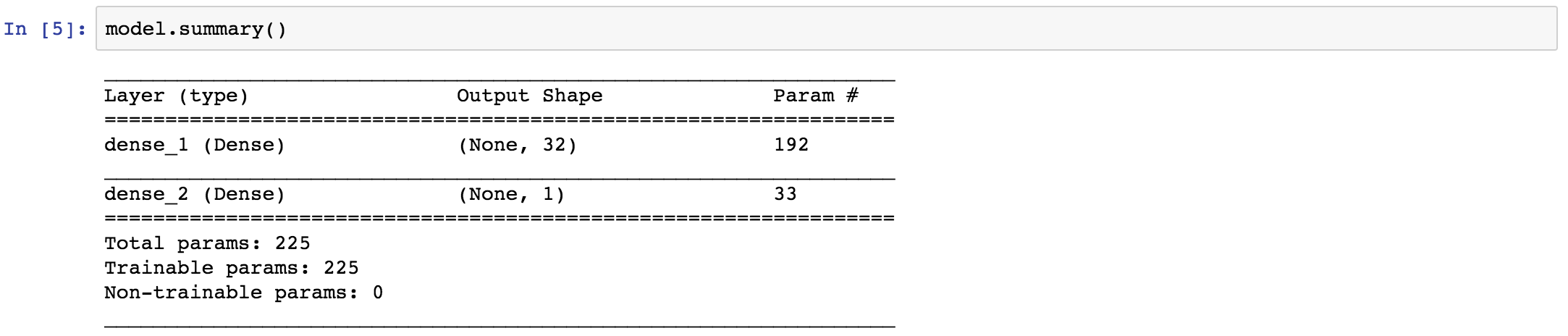Figure 1.4: Example of a Keras model summary

1. Training the model is straightforward with one command, while simultaneously saving the results to a variable called history:
history = model.fit(x_input, y_input, epochs=10, batch_size=32)
1. For testing, the prediction function can be used after training:
pred = model.predict(x_input, batch_size=128)

Note

In this short introduction to Keras, we have demonstrated how easy it is to implement a neural network in just a couple of lines of code. However, don't confuse simplicity with power. The Keras framework provides much more than we've just demonstrated here and one can adjust their model up to a granular level if needed.

Using PyTorch’s dynamic computation graphs for RNNs

PyTorch is the Python deep learning framework and it's getting a lot of traction lately. PyTorch is the implementation of Torch, which uses Lua. It is by Facebook and is fast thanks to GPU-accelerated tensor computations. A huge benefit of using over other frameworks is that graphs are created on the fly and are not static. This means networks are dynamic and you can adjust your network without having to start over again. As a result, the graph that is created on the fly can be different for each example. PyTorch supports multiple GPUs and you can manually set which computation needs to be performed on which device (CPU or GPU).

How to do it...

1. First, we install in our Anaconda environment, as follows:
conda install pytorch torchvision cuda80 -c soumith

If you want to install on another platform, you can have a look at the PyTorch website for clear guidance: http://pytorch.org/.

1. Let's import PyTorch into our Python environment:
import torch
1. While Keras provides higher-level abstraction for building neural networks, PyTorch has this feature built in. This means one can build with higher-level building blocks or can even build the forward and backward pass manually. In this introduction, we will use the higher-level abstraction. First, we need to set the size of our random training data:
batch_size = 32
input_shape = 5
output_shape = 10
1. To make use of GPUs, we will cast the tensors as follows:
torch.set_default_tensor_type('torch.cuda.FloatTensor')

This ensures that all computations will use the attached GPU.

1. We can use this to generate random training data:
X = Variable(torch.randn(batch_size, input_shape))
1. We will use a simple neural network having one hidden layer with 32 units and an output layer:
model = torch.nn.Sequential(
torch.nn.Linear(input_shape, 32),
torch.nn.Linear(32, output_shape),
).cuda()

We use the .cuda() extension to make sure the model runs on the GPU.

1. Next, we the MSE loss function:
loss_function = torch.nn.MSELoss()
1. We are now ready to start training our model for 10 epochs with the following code:
learning_rate = 0.001
for i in range(10):
y_pred = model(x)
loss = loss_function(y_pred, y)
print(loss.data)
loss.backward()

# Update weights
for param in model.parameters():

Note

The PyTorch framework gives a lot of freedom to implement simple neural networks and more complex deep learning models. What we didn't demonstrate in this introduction, is the use of dynamic graphs in PyTorch. This is a really powerful feature that we will demonstrate in other chapters of this book.

Implementing high-performance models with CNTK

Microsoft also introduced its open source deep framework not too long ago: Microsoft Cognitive Toolkit. This framework is better known as CNTK. is written in C++ for performance reasons and has a Python API. CNTK supports GPUs and multi-GPU usage.

How to do it...

1. First, we install CNTK with pip as follows:
pip install https://cntk.ai/PythonWheel/GPU/cntk-2.2-cp35-cp35m-linux_x86_64.whl

Adjust the wheel file if necessary (see https://docs.microsoft.com/en-us/cognitive-toolkit/Setup-Linux-Python?tabs=cntkpy22).

1. After installing CNTK, we can import it into our Python environment:
import cntk
1. Let's create some simple dummy data that we can use for training:
import numpy as np
x_input = np.array([[1,2,3,4,5]], np.float32)
y_input = np.array([], np.float32)
1. Next, we need to define the placeholders for the input data:
X = cntk.input_variable(5, np.float32)
y = cntk.input_variable(1, np.float32)
1. With CNTK, it's straightforward to stack multiple layers. We stack a dense layer with 32 inputs on top of an output layer with 1 output:
from cntk.layers import Dense, Sequential
model = Sequential([Dense(32),
Dense(1)])(X)
1. Next, we define the loss function:
loss = cntk.squared_error(model, y)
1. Now, we are ready to finalize our model with an optimizer:
learning_rate = 0.001
1. Finally, we can train our model as follows:
for epoch in range(10):
trainer.train_minibatch({X: x_input, y: y_input})

Note

As we have demonstrated in this introduction, it is straightforward to build models in CNTK with the appropriate high-level wrappers. However, just like TensorFlow and PyTorch, you can choose to implement your model on a more granular level, which gives you a lot of freedom.

Building efficient models with MXNet

The MXNet deep learning framework you to build efficient deep learning models in Python. Next to Python, it also let you build models in popular languages as R, Scala, and Julia. Apache MXNet is supported by Amazon and Baidu, amongst others. MXNet has proven to be fast in benchmarks and it supports GPU and multi-GPU usages. By using lazy evaluation, MXNet is able to automatically execute operations in parallel. Furthermore, the MXNet frameworks uses a symbolic interface, called Symbol. This simplifies building neural network architectures.

How to do it...

1. To install MXNet on Ubuntu with GPU support, we can use the command in the terminal:
pip install mxnet-cu80==0.11.0

For other platforms and non-GPU support, have a look at https://mxnet.incubator.apache.org/get_started/install.html.

1. Next, we are ready to import mxnet in our Python environment:
importmxnetasmx
1. We create some simple dummy data that we assign to the GPU and CPU:
import numpy as np
x_input = mx.nd.empty((1, 5), mx.gpu())
x_input[:] = np.array([[1,2,3,4,5]], np.float32)

y_input = mx.nd.empty((1, 5), mx.cpu())
y_input[:] = np.array([[10, 15, 20, 22.5, 25]], np.float32)
1. We can easily copy and adjust the data. Where possible MXNet will automatically execute operations in parallel:
x_input
w_input = x_input
z_input = x_input.copyto(mx.cpu())
x_input += 1
w_input /= 2
z_input *= 2
1. We can print the output as follows:
print(x_input.asnumpy())
print(w_input.asnumpy())
print(z_input.asnumpy())
1. If we want to feed our data to a model, we should create an iterator first:
batch_size = 1
train_iter = mx.io.NDArrayIter(x_input, y_input, batch_size, shuffle=True, data_name='input', label_name='target')
1. Next, we can create the symbols for our model:
X = mx.sym.Variable('input')
Y = mx.symbol.Variable('target')
fc1 = mx.sym.FullyConnected(data=X, name='fc1', num_hidden = 5)
lin_reg = mx.sym.LinearRegressionOutput(data=fc1, label=Y, name="lin_reg")
1. Before we can start training, we need to define our model:
model = mx.mod.Module(
symbol = lin_reg,
data_names=['input'],
label_names = ['target']
)
1. Let's start training:
model.fit(train_iter,
optimizer_params={'learning_rate':0.01, 'momentum': 0.9},
num_epoch=100,
batch_end_callback = mx.callback.Speedometer(batch_size, 2))
1. To use the trained model for prediction we:
model.predict(train_iter).asnumpy()

Note

We've shortly introduced the MXNet framework. In this introduction, we've demonstrated how easily one can assign variables and computations to a CPU or GPU and how to use the Symbol interface. However, there is much more to explore and the MXNet is a powerful framework for building flexible and efficient deep learning models.

Defining networks using simple and efficient code with Gluon

The newest addition to the range of deep learning frameworks is Gluon. Gluon is recently launched by AWS and Microsoft to provide an API simple, easy-to-understand code without the loss of performance. Gluon is already included in the latest release of MXNet and will be available in future releases of CNTK (and other frameworks). Just like Keras, Gluon is a wrapper around other deep learning frameworks. The main difference between Keras and Gluon, is that Gluon will (at first) focus on imperative frameworks.

How to do it...

1. At the moment, gluon is included in the latest release of MXNet (follow the steps in Building efficient models with MXNet to install MXNet).
2. After installing, we can directly import gluon as follows:
from mxnet import gluon
1. Next, we create some dummy data. For this we need the data to be in MXNet's NDArray or Symbol:
import mxnet as mx
import numpy as np
x_input = mx.nd.empty((1, 5), mx.gpu())
x_input[:] = np.array([[1,2,3,4,5]], np.float32)

y_input = mx.nd.empty((1, 5), mx.gpu())
y_input[:] = np.array([[10, 15, 20, 22.5, 25]], np.float32)
1. With Gluon, it's really straightforward to build a neural network by stacking layers:
net = gluon.nn.Sequential()
with net.name_scope():
1. Next, we initialize the parameters and we store these on our GPU as follows:
net.collect_params().initialize(mx.init.Normal(), ctx=mx.gpu())
1. With the following code we set the loss function and the optimizer:
softmax_cross_entropy = gluon.loss.SoftmaxCrossEntropyLoss()
trainer = gluon.Trainer(net.collect_params(), 'adam', {'learning_rate': .1})
1. We're ready to start training or model:
n_epochs = 10

for e in range(n_epochs):
for i in range(len(x_input)):
input = x_input[i]
target = y_input[i]
output = net(input)
loss = softmax_cross_entropy(output, target)
loss.backward()
trainer.step(input.shape)

Note

We've shortly demonstrated how to implement a neural network architecture with Gluon. Gluon is a powerful extension that can be used to implement deep learning architectures with clean code. At the same time, there is almost no performance loss when using Gluon.